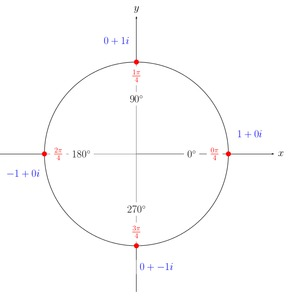# Complex Cube and Fourth Roots of 1

Alignments to Content Standards: N-CN.A.2 N-CN.B.5 N-CN.C.8

For each odd positive integer $n$, the only real number solution to $x^n = 1$ is $x = 1$ while for even positive integers $n$, $x = 1$ and $x = -1$ are solutions to $x^n = 1$. In this problem we look for all complex number solutions to $x^n = 1$ for some small values of $n$.

1. Find all complex numbers $a + bi$ whose cube is 1.
2. Find all complex numbers $a + bi$ whose fourth power is 1.

## IM Commentary

This task has students further their understanding of the algebra of the complex numbers. It is easy to get the impression that the complex numbers are the real numbers plus a solution to $x^2=-1$, leaving many other algebraic equations without solutions. In fact, the opposite is true -- the set of complex numbers contains (among other things) $n$-th roots of all real numbers. The task asks students to explicitly identify two examples of such roots, the complex cube and fourth roots of 1. Three different solutions are presented, focusing respectively on:

• Factoring the polynomials $x^3-1=0$ and $x^4-1=0$.
• The geometric approach to multiplication of complex numbers.
• Direct algebraic manipulations to solve $(a+bi)^3=1$ and $(a+bi)^4=1$.

As such, there are many options for implementation in the classroom. The task could be used as an illustration of any of the three methods defined above, or as a more open-ended scenario where students might independently choose one of the three (or others!), illustrating the Standard for Mathematical Practice MP 5 (Use appropriate tools strategically). In this latter option, it may be profitable to have students work in small groups to brainstorm solution techniques on their own, sharing these techniques in a whole-class discussion afterwards.

## Solutions

Solution: 1 Factoring a polynomial

1. We are trying to find solutions to the equation $x^3 = 1$ which are the roots of the equation $x^3-1=0$. One solution is $x = 1$. This means that $x-1$ divides $x^3 -1$ with no remainder. Performing long division, we find $$x^3-1 = (x-1)(x^2+x+1).$$ To find the roots of the polynomial $x^2 +x+ 1$ we can use the quadratic formula which says that the roots are $$\frac{-1 \pm \sqrt{1-4}}{2} = \frac{-1}{2} \pm \frac{\sqrt{-3}}{2}.$$ We know that $i^2 = -1$ so $(\sqrt{3}i)^2 = -3$ and this means that $\sqrt{-3} = \sqrt{3}i$. So our two roots of $x^2 - x + 1$ are $$-\frac{1}{2} \pm \frac{\sqrt{3}}{2}i$$

We have found 3 solutions to $x^3-1 = 0$, namely $x = 1, x = -\frac{1}{2} + \frac{\sqrt{3}i}{2},$ and $x = -\frac{1}{2} - \frac{\sqrt{3}i}{2}$.

2. We can apply the same technique to solve the equation $x^4 = 1$ or $x^4 - 1 = 0$. We can first write $x^4 -1$ as a difference of squares: $$x^4-1 = (x^2 + 1)(x^2 - 1).$$ We have $x^2 -1 = (x+1)(x-1)$. Similarly we have $x^2 + 1 = (x+i)(x-i)$. So this gives $$x^4 + 1 = (x+i)(x-i)(x+1)(x-1)$$ and the solutions to $x^4-1 = 0$ are $x = -i,i,-1,1$.

Solution: 2 Using Geometry

Suppose $z$ is a complex number which can be written in polar form as follows: $$z = re^{i\theta}.$$ Here $r$ represents the distance from $z$ to 0 and $\theta$ is the measure of the directed angle made by the non-negative real numbers and the ray from 0 through $z$. One important property of the polar form is that it facilitates calculating powers:

\begin{align} z^n &= \left(re^{i\theta}\right)^n \\ &= r^ne^{in\theta}. \end{align}
1. Using the polar form for a complex number $z$ and its properties indicated above, $z = re^{i\theta}$ is a cube root of 1 when $z^3 = 1$ or $$r^3e^{i3\theta} = 1.$$ This means that $r^3 = 1,$ so $r = 1$ since $r$ is a real number and $r\geq0$. Moreover $3\theta$ is an integer multiple of $2\pi$. This means we can have $\theta = 0$, $\theta = \frac{2\pi}{3}$, or $\theta = \frac{4\pi}{3}$. These are the solutions for $\theta$ that we find by choosing our integer multiple of $\pi$ to be 0, 1, and 2. If we choose different integer multiples we will find angles that differ from the three listed above by an integer multiple of 2$\pi$. The three cube roots of 1 are pictured below:Notice that the three numbers whose cube is 1 evenly divide the unit circle into 3 equal pieces.

2. Writing $z = re^{i\theta}$, the solutions of $z^4 = 1$ are the same as the solutions to $$r^4e^{i4\theta} = 1.$$ This means that $r^4 = 1,$ so $r=1$ since $r$ is a real number and $r \geq 0$. In addition $4\theta$ must be an integer multiple of $2\pi$. Choosing multiples of 0, 1, 2, and 3 gives $\theta= 0, \frac{\pi}{2}, \pi, \frac{3\pi}{2}$. These correspond to the four numbers 1, $i$, -1, and $-i$, as pictured below:The fourth roots of 1 divide the unit circle evenly into four sections.

Solution: 3 Solving equations

1. Writing $(a+bi)^3=(a^3-3ab^2)+(3a^2b-b^3)i=1+0i$ and equating real and imaginary parts of $(a+bi)^3=1$ gives $$a^3 - 3ab^2 = 1$$ and $$3a^2b - b^3 = 0$$ Factoring the second equation as $b(3a^2-b^2)=0$, we see that either $b=0$ or $3a^2=b^2$. If $b=0$, then $a^3=1$, giving the obvious cube root of 1. If $b\neq 0$, then $3a^2=b^2$, and substituting this into $a^3-3ab^2=1$ gives $a^3-9a^3=1$, so $a=-\frac{1}{2}$, and then $b=\pm\frac{\sqrt{3}}{2}$.
2. Similarly, if we write $$(a+bi)^4=((a^2-b^2)+2abi)^2= (a^4-6a^2b^2+b^4)+(4a^3b-4ab^3)i$$ then equating imaginary parts in $(a+bi)^4=1$, gives $$(4a^3b-4ab^3)=0.$$ Factoring the left-hand side as $4ab(a^2-b^2)=0$, we see that any 4th root of 1 must have $a=0$, $b=0$, or $a^2=b^2$. If $a=0$, then the equation $(bi)^4=1$ quickly gives $b=\pm 1$. Similarly, if $b=0$, then the equation $a^4=1$ again gives $a=\pm 1$ (remember that $a$ and $b$ are real). Finally, if $a=\pm b$, then equating the real parts of $(a\pm ai)^4=1$ gives $$(a^4-6a^2a^2+a^4)=1$$ or $-4a^4=1$. Since there are no real values of $a$ for which this is true, the only solutions to these equations are $(a,b)=(\pm 1,0)$ and $(a,b)=(0,\pm 1)$, corresponding to the four roots $\pm 1$ and $\pm i$.